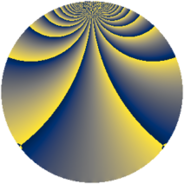# Properties

 Label 1470.2.yLevel $1470$ Weight $2$ Character orbit 1470.y Rep. character $\chi_{1470}(263,\cdot)$ Character field $\Q(\zeta_{12})$ Dimension $320$ Sturm bound $672$

# Related objects

## Defining parameters

 Level: $$N$$ $$=$$ $$1470 = 2 \cdot 3 \cdot 5 \cdot 7^{2}$$ Weight: $$k$$ $$=$$ $$2$$ Character orbit: $$[\chi]$$ $$=$$ 1470.y (of order $$12$$ and degree $$4$$) Character conductor: $$\operatorname{cond}(\chi)$$ $$=$$ $$105$$ Character field: $$\Q(\zeta_{12})$$ Sturm bound: $$672$$

## Dimensions

The following table gives the dimensions of various subspaces of $$M_{2}(1470, [\chi])$$.

Total New Old
Modular forms 1472 320 1152
Cusp forms 1216 320 896
Eisenstein series 256 0 256

## Trace form

 $$320q - 8q^{6} + O(q^{10})$$ $$320q - 8q^{6} - 4q^{10} + 24q^{15} + 160q^{16} + 16q^{18} + 40q^{22} + 16q^{25} + 72q^{27} + 12q^{30} - 32q^{31} + 20q^{33} + 40q^{36} + 16q^{37} + 64q^{43} + 28q^{45} - 24q^{46} + 16q^{55} - 40q^{57} + 28q^{58} - 16q^{60} - 24q^{61} + 16q^{67} - 16q^{72} + 56q^{73} - 8q^{75} + 32q^{76} + 32q^{78} - 12q^{81} - 16q^{82} - 16q^{85} - 76q^{87} - 20q^{88} - 40q^{90} - 116q^{93} - 4q^{96} - 104q^{97} + O(q^{100})$$

## Decomposition of $$S_{2}^{\mathrm{new}}(1470, [\chi])$$ into newform subspaces

The newforms in this space have not yet been added to the LMFDB.

## Decomposition of $$S_{2}^{\mathrm{old}}(1470, [\chi])$$ into lower level spaces

$$S_{2}^{\mathrm{old}}(1470, [\chi]) \cong$$ $$S_{2}^{\mathrm{new}}(105, [\chi])$$$$^{\oplus 4}$$$$\oplus$$$$S_{2}^{\mathrm{new}}(210, [\chi])$$$$^{\oplus 2}$$$$\oplus$$$$S_{2}^{\mathrm{new}}(735, [\chi])$$$$^{\oplus 2}$$